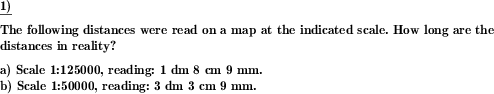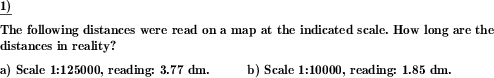Custom math worksheets at your fingertips# Details for problem "Convert the value of a scaled length"

Quickname: 2050

Elementary School, Primary School, Junior High School, Middle School.

## Summary

Conversion of lengths at a given scale in both directions

## Examples## Description

Lengths are to be converted at a given scale.

The scale is selectable in two ranges. Furthermore, it must be specified in which direction (model->reality or, reality->model) the conversion is to be made. Scales are used on maps to measure reality distances. This worksheet is used to convert values of a scaled length to reality and vice versa.

Practicing these worksheets will buildup the following skills.

• Apply multiplication and division concepts while converting the values.
• Revise the concept of reading scales according to set values.
• Conversion of measures will be exercised.
• Reading scales and converting between units of measure with more confidence, will be achieved.
• Decimal numbers exercise will be done.
• Grasp the skill to use different units of measurement accordingly.

These worksheets will boost critical thinking and problem-solving skills in the context of real-life problems. In each question, a configurable number of length scales with values are given. Conversion is to be done for each scale.

The number of problems, scaling factor, direction of conversion, and notation of length specification can be chosen. Each problem can be generated with problems starting from 1 problem and a maximum of 10 problems per worksheet. The scaling factor is up to 500000. Conversion can be done in both ways, model to reality and reality to model. Notation of length can also vary in multiple unit steps, in decimal notation, and whole numbers only.

The answer key will provide the converted solution with units.

Download free printable worksheets for this math problem here. The worksheet contains the problems only, the solution sheet includes the answers. Just click on the respective link.

•Worksheet 1Solution sheet with answers
•Worksheet 2Solution sheet with answers
•Worksheet 3Solution sheet with answers

If you can not see the solution sheets for download, they may be filtered out by an ad blocker that you may have installed. If this is the case, please allow ads for this page and reload the page. The solution sheets will then reappear.

• Do these sample worksheets do not really fit?
• Do you need more math worksheets, with a different level of difficulty?
• Would you like to combine different problems on a worksheet and adjust them to your needs?
• As a teacher, you can put together your own worksheets using the automatically generated math problems provided.
With a free initial credit, you can start creating your own math worksheets in a few minutes.

You can try it for free! Register here, to create custom worksheets now!

## Customization options for this problem

Parameter
Possible values
Number of problems
1, 2, 3, 4, 5, 6, 7, 8, 9, 10
Scaling factor
1:5-1:100, 1:10000-1:500000
Direction of conversion
model->reality, reality->model
Notation of length specification
in multiple unit steps, in decimal notation, whole numbers only

## Similar problems

Remark
Description
In tabular form: fill empty space for scale factor, real or mapped length
In a table with scale, scaled length and real length, missing values must be added
Only the scale factor has to be determined
The scale used for an illustration shall be determined.